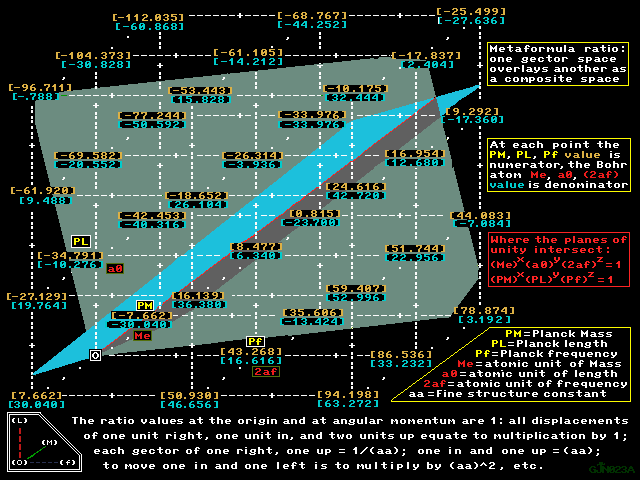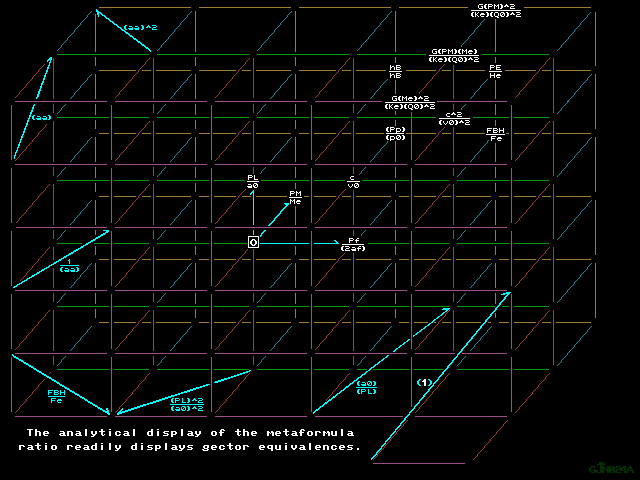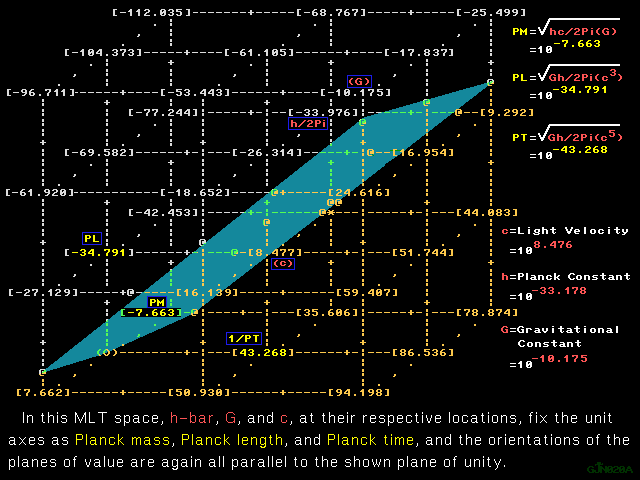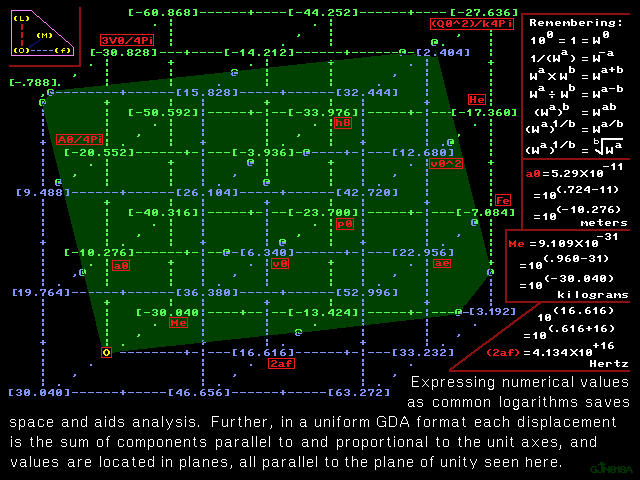```Color print      1280 X 960 GIF      Grayscale print

Tutorial back     Values table       Symbol key     Tutorial nextColor print      1280 X 960 GIF      B & W print

METAFORMULA RATIO

The two graphics above, one numerical and one analytical, each show
the same abstract space, the metaformula ratio of the two differing
spaces in the two graphics below: the Planck unit space of GJN020A
immediately below is overlaid on the atomic unit space of GJN018A
appearing last, and at every point in each composite map above, the
numerical value is the ratio of the Planck unit quantity of GJN020A
as numerator to the atomic unit quantity of GJN018A as denominator.
Since the units cancel in each ratio, any point in either composite
space represents a dimensionless number, and every vector in either
composite space represents the statement of numerical relationship
between the dimensionless ratios at its endpoints.Color print      1280 X 960 GIF      Grayscale printColor print      1280 X 960 GIF      Grayscale print

```